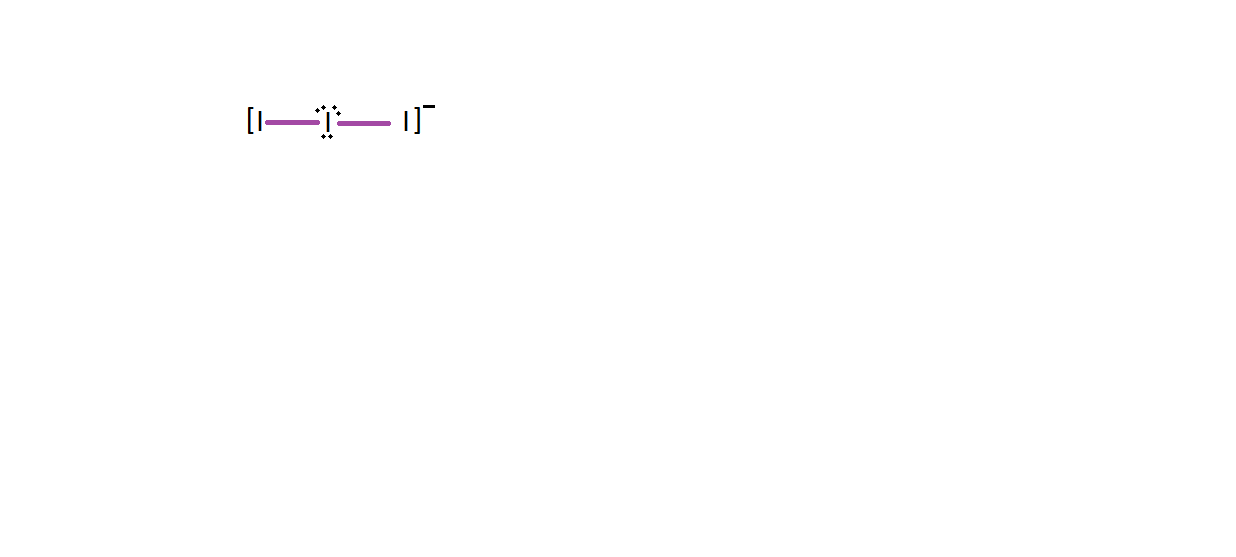Courses
Courses for Kids
Free study material
Free LIVE classes
MoreLIVE
Join Vedantu’s FREE Mastercalss

# How many bonding pairs and lone pairs surround the central atom in the $\text{I}_{3}^{-}$ ion?A. 2, 2B. 2, 3C. 3, 2D. 4, 3Verified
334.5k+ views
Hint: Hybridisation is the mixing of an atomic orbital to form the chemical bonds according to the valence band theory. It forms a new orbital with the different geometry and shape of the hybridised molecule.

-Firstly, we will calculate the hybridisation by using the formula that is
$\text{H = }\dfrac{1}{2}(\text{V + M +C - A)}$
-Here, H is the hybridisation, V is the valence electron of the central atom, M is the monovalent atom that is linked to the central atom, C is the charge present on the cation and A is the charge present on the anion.
-Now, we know that there are a total of 7 valence electrons in the iodine and it is a monovalent atom also.
-So, the value of V and M for iodine will be 7 and 2 respectively because two 2 molecules of iodine are linked to the central molecule of iodine.
-The value of C will be 1 because there is only a -1 charge on the iodine molecule.
-So, the value of H is:
$\text{H = }\dfrac{1}{2}(7\text{ }+\text{ }2\text{ + 1)}$
$\text{H = }\dfrac{1}{2}\text{ }\cdot \text{ 10 = 5}$
-The hybridisation of the centre iodine will be $\text{s}{{\text{p}}^{3}}\text{d}$ and it will have 3 lone pairs and 2 bond pairs with a linear shape.
-Central iodine makes 2 bonds and the 3 pairs remain unused.
-So, they arrange themselves such that there is minimum repulsion between the lone pairsSo, the correct answer is “Option B”.

Note: VSEPR or Valence Shell Electron Pair Theory tells us about the geometry of a molecule by arranging the molecules in such a way that there will be less repulsion between them.
Last updated date: 24th Sep 2023
Total views: 334.5k
Views today: 4.34k